## Monday, January 25, 2016

### Productive Struggle: Volume

I handed each student a bags of 40 cubes and asked each students to create a rectangle prism using all the cubes. Students started building. There were many questions and lots of trial and error. Few realized that length times width times height equal volume. Most started building before thinking about the numbers involved.

It took the students a long time to build. The cubes were somewhat difficult to connect, but it was also clear that they haven't done this much. In time, however, we had many different rectangular prisms with volumes equal to 40 cubic units.

We discussed the project beginning first with naming the properties of a cube. That helped students to identify the important language and concepts involved. Students will revisit those concepts and words again later in the week when they build a number of solid figures from nets. Then students shared their creations as I drew a picture of each one on the board and listed the dimensions.

Tomorrow we'll use our POWerful SRSD problem solving approach to solve a multi-step volume problem. Later in the year we'll study volume with greater depth, but for now, these activities provide a good start.

Note: It is important to define prism for this problem. This information comes from Math is Fun

polyhedron is a solid with flat faces
(from Greek poly- meaning "many" and -edron meaning "face").
Each face is a polygon (a flat shape with straight sides).
So: no curved surfaces!

## Examples of Polyhedra:

Cube Triangular Prism Dodecahedron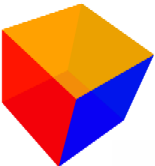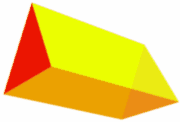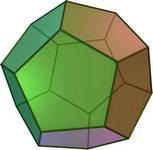Its faces are all squares Its faces are trianglesand rectangles What faces does it have?

## Common Polyhedra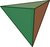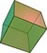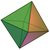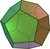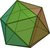Platonic Solids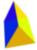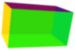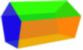Prisms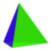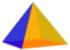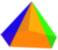Pyramids

## Many More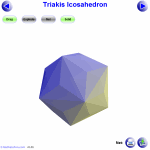Explore 100s of Animated Polyhedron Models. You can also see some Images of Polyhedra if you want.

## Counting Faces, Vertices and Edges

When we count the number of faces (the flat surfaces), vertices (corner points), and edges of a polyhedron we discover an interesting thing:
The number of faces plus the number of vertices
minus the number of edges equals 2
This can be written neatly as a little equation:

### F + V − E = 2

It is known as Euler's Formula (or the "Polyhedral Formula") and is very useful to make sure we have counted correctly!

### Let's try some examples:

 This cube has: 6 Faces 8 Vertices (corner points) 12 EdgesF + V − E = 6 + 8 − 12 = 2

 This prism has: 5 Faces 6 Vertices (corner points) 9 EdgesF + V − E = 5 + 6 − 9 = 2
But there are cases where it does not work! Read Euler's Formula for more.